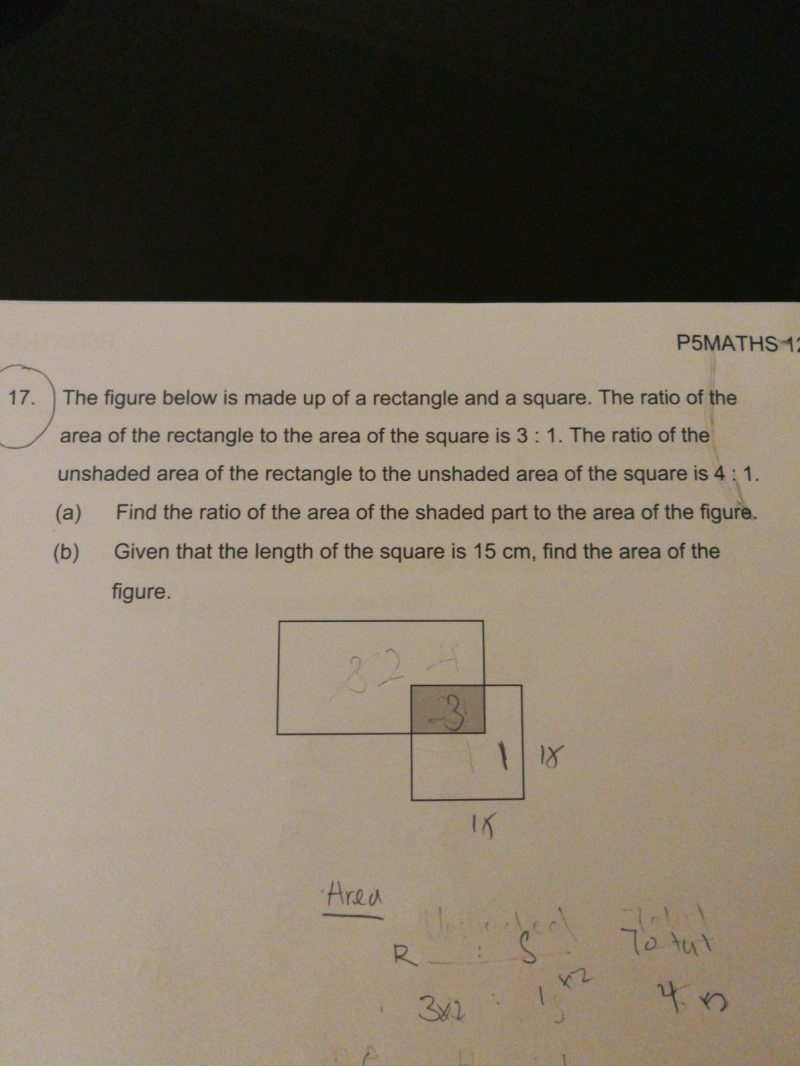# QuestionHi,

Can someone help on the above question?

Thanks

(a)
rectangle : square
3 : 1
9 : 3

unshaded area of rectangle : unshaded area of square : shaded area : total area of figure
4 : 1 : ? : ?
8 : 2 : 1 : 11

ratio of the area of the shaded part to the area of the figure ——-> 1 : 11

(b)
15 x 15 = 225 (area of square)
225 x 3 = 675 (area of rectangle)
225 + 675 = 1000
1000/11 = 90 10/11 (area of shaded part)
1000 – 90 10/11 = 909 1/11

Ans : (a) 1 : 11; (b) 909 1/11 sq cm.

0 Replies 0 Likes

Rect : Square = 3 : 1

Thence

and

Rect = 3 x Square   same as

Thence

thence

Let   shaded is 1 unit, then

Total is 11 units

Sorry, I’m very confused with your explaination. Can explain using ratio or model units so that I can show my DD ?

Thanks

Sorry, didn’t check my email.

Let me try to draw:

unshaded-rect : unshaded-square = 4:1  ( use U for one unit( or you can draw a shape))

U U U U : U

U U U Us: Us = 3:1

we know

Us Us Us : Us = 3:1

then U U U Us = Us Us Us

then U U U = Us Us

cancel two U both sides, then U = s s

If you want me to draw, try here mathecamp.mathawt.com/ask_question

4:1 =>

U U U U

U

3:1

U U U Us

Us

or draw like this( turn one U into  two s)

Us Us U Us

0 Replies 0 Likes